# Bayesian approach

to statistical problems

An approach based on the assumption that to any parameter in a statistical problem there can be assigned a definite probability distribution. Any general statistical decision problem is determined by the following elements: by a space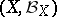of (potential) samples, by a spaceof values of the unknown parameter, by a family of probability distributionson, by a space of decisions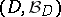and by a function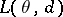, which characterizes the losses caused by accepting the decision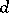when the true value of the parameter is. The objective of decision making is to find in a certain sense an optimal rule (decision function)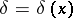, assigning to each result of an observationthe decision. In the Bayesian approach, when it is assumed that the unknown parameteris a random variable with a given (a priori) distribution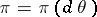onthe best decision function (Bayesian decision function)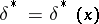is defined as the function for which the minimum expected loss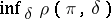, where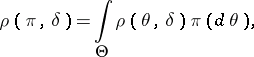and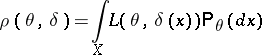is attained. Thus,In searching for the Bayesian decision function, the following remark is useful. Let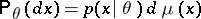,, whereandare certain-finite measures. One then finds, assuming that the order of integration may be changed,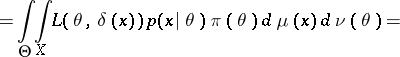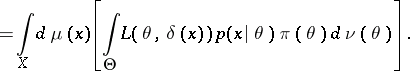It is seen from the above that for a givenis that value of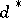for which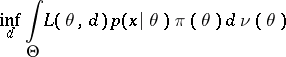is attained, or, what is equivalent, for which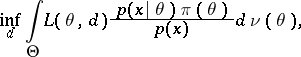is attained, where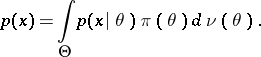But, according to the Bayes formula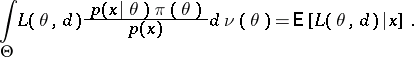Thus, for a given,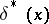is that value offor which the conditional average lossattains a minimum.

Example. (The Bayesian approach applied to the case of distinguishing between two simple hypotheses.) Let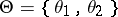,,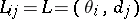,;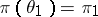,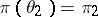,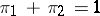. If the solution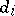is identified with the acceptance of the hypothesis:, it is natural to assume that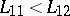,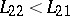. Then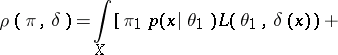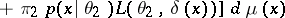implies thatis attained for the function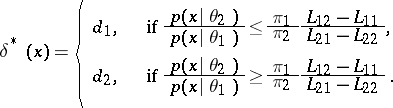The advantage of the Bayesian approach consists in the fact that, unlike the losses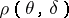, the expected losses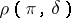are numbers which are dependent on the unknown parameter, and, consequently, it is known that solutions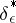for which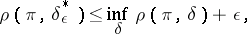and which are, if not optimal, at least-optimal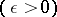, are certain to exist. The disadvantage of the Bayesian approach is the necessity of postulating both the existence of an a priori distribution of the unknown parameter and its precise form (the latter disadvantage may be overcome to a certain extent by adopting an empirical Bayesian approach, cf. Bayesian approach, empirical).